# Range in Algebra

Range in Algebra

The range of a function in algebra is the set of all its outputs. Consider a situation where you are asked to find the cubes of the first 10 natural numbers. The values 1, 8, 27, 64, 125, 216, 343, 512, 729, and 1000 are the set of outputs that are called the range.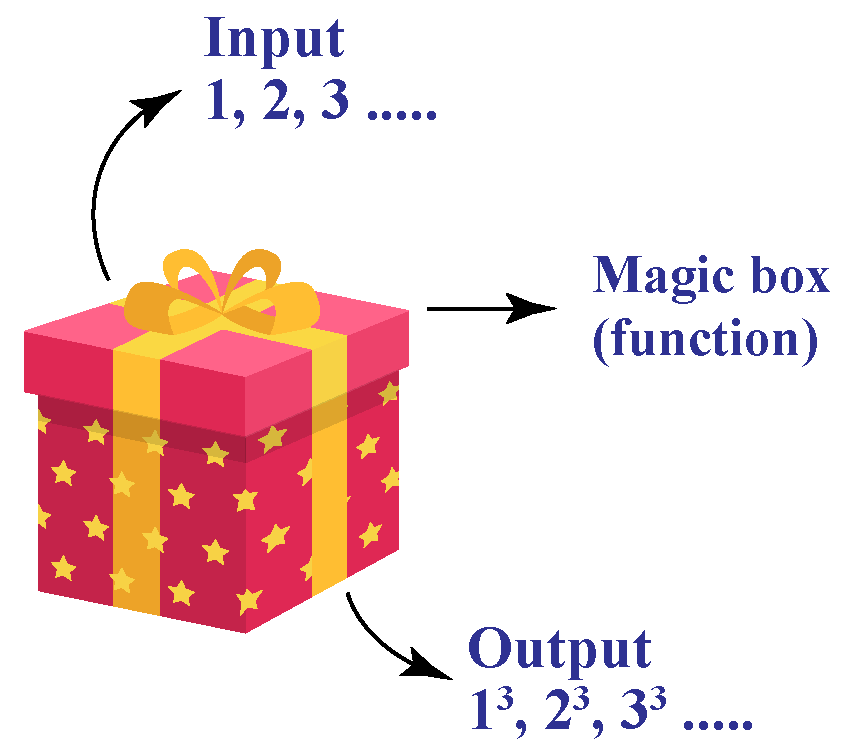In this chapter, once you have understood the basics of range in algebra, you will learn how to find the range of a function, examples of domain and range, and how to find the range of a function.

Check out the interactive examples to know more about the lesson and try your hand at solving a few interesting practice questions at the end of the page.

## Lesson Plan

 1 What is Meant By Range in Algebra? 2 Tips and Tricks 3 Solved Examples on Range in Algebra 4 Important Notes on Range in Algebra 5 Interactive Questions on Range in Algebra

## What is Meant By Range in Algebra?

The range of a function is the set of all its outputs.

Example:

Let us consider the function $$f:\,\, A \rightarrow A$$, where $$A= \{1,2,3,4\}$$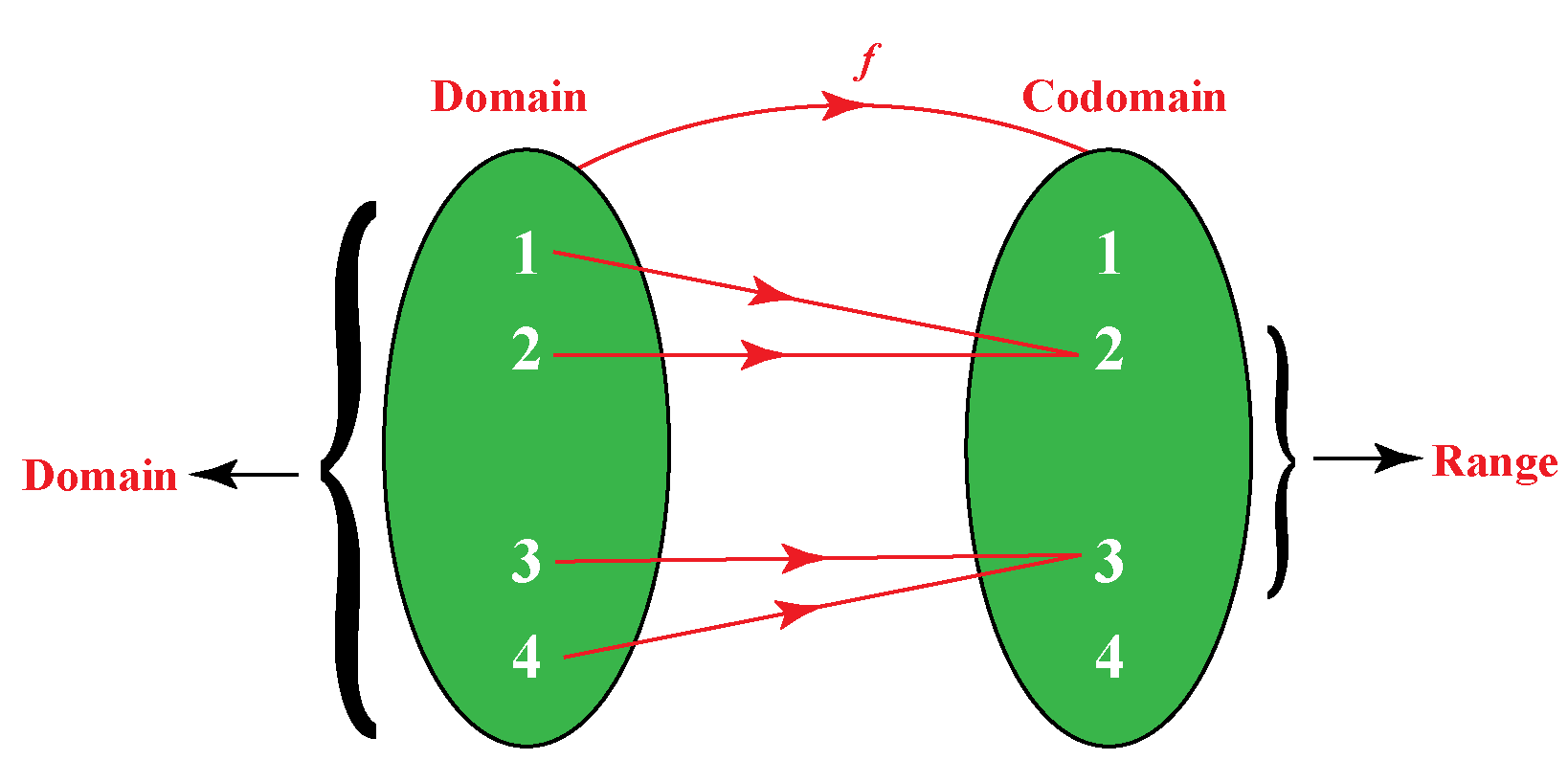The elements of the domain are called pre-images and the elements of the codomain which are mapped are called the images.

Here, the range of the function $$f$$ is the set of all images of the elements of the domain (or) the set of all the outputs of the function.

Thus, the range of $$f$$ = $$\{2, 3\}$$

## How to Find the Range of a Function in Algebra?

Let's learn the process of finding the ranges of different types of functions.

### Range of a Quadratic Function

Before learning the process of finding the range of a quadratic function, $$f(x)=ax^2+bx+c$$, let's recall a few points.

• The quadratic function has a minimum value if $$a>0$$
• The quadratic function has a maximum value if $$a<0$$

Let's take an example of a quadratic function.

Example

Find the range of the quadratic function $$y=x^{2}+6 x+8$$

Solution

To find the range of the given quadratic function, first, we need to convert it to the vertex form by completing the square.

Then we get:

\begin{align} y &= x^{2}+6 x+8\\ &= (x^2+6x)+8\\ &= (x^2+6x+9)-9+8\\ &= (x+3)^2 -1 \end{align}

Comparing this with the vertex form $$a(x-h)^2+k$$, we get

\begin{align} a &=1 \\ h &=-3\\ k &=-1 \end{align}

So the vertex is $$(h,k)=(-3, -1)$$

Since $$a=1>0$$, the quadratic function has a minimum value.

The minimum value of the quadratic function is the y-coordinate of the vertex, which is -1

Thus, the range of the given quadratic function is:

 $$\{y \in R / y \geq -1\}$$ (OR) $$[-1, \infty)$$

We can find the range by graphing the function as well. After graphing, we just look for the values on the y-axis that are covered by the graph.Here, $$R$$ is the set of all real numbers.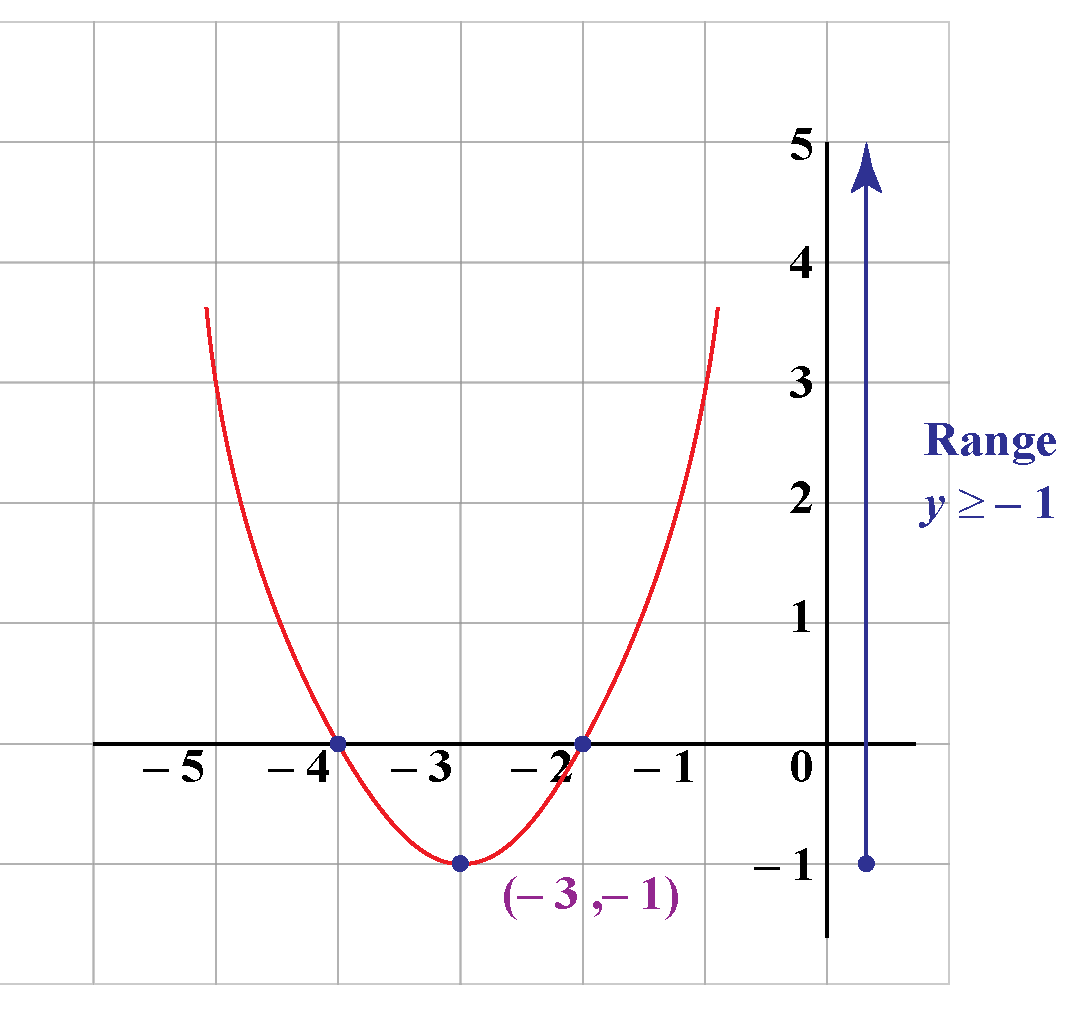### Range of a Rational Function

To find the range of a rational function of the form $$y = \dfrac{ax+b}{bx+c}$$,

• We solve the function for $$x$$.
• We find the range by finding the excluded values of the resultant equation.

Example

Find the range of $$y=\dfrac{x}{2-4 x}$$

Solution

Let us solve the given equation for $$x$$

\begin{align} (2-4x)y &= x\\[0.2cm] 2y-4xy &=x\\[0.2cm] 2y&=x+4xy\\[0.2cm] x(1+4y) &= 2y\\[0.2cm] x &= \dfrac{2y}{1+4y} \end{align}

The final equation is a fraction and a fraction is NOT defined when its denominator is zero. So

\begin{align}1+4y &\neq 0 \\[0.2cm] 4y &\neq -1\\[0.2cm] y &\neq \dfrac{-1}{4} \end{align}

Therefore, the range of the given function is,

 $$\left\{ y \in R/y \neq \dfrac{-1}{4} \right\}$$ (OR) $$\left(-\infty, \dfrac{-1}{4}\right) \cup \left(\dfrac{-1}{4}, \infty\right)$$

Let's calculate the range of the given function by graphing it.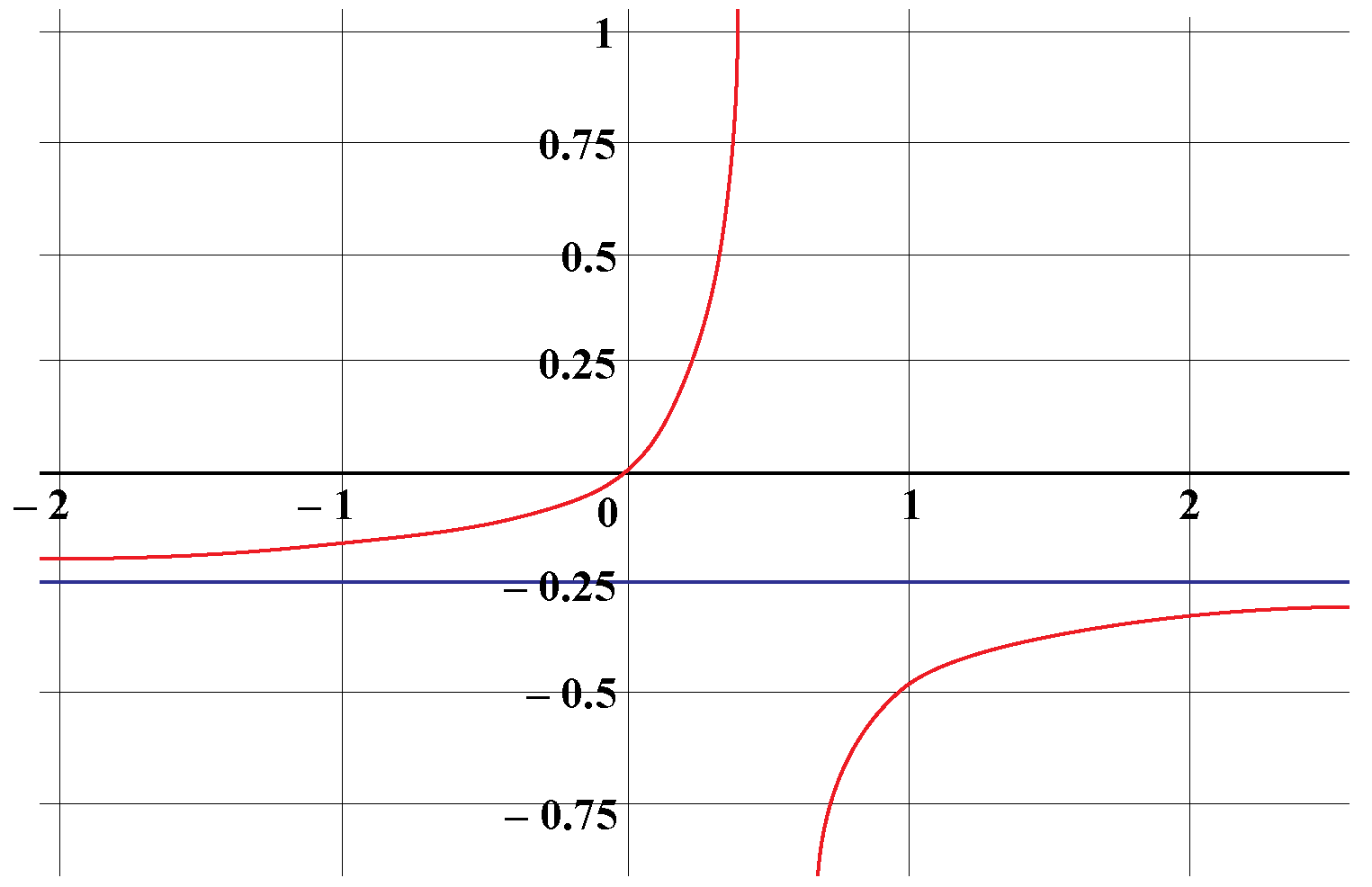Here, we can see that all the values on the y-axis, except $$y=-0.25$$, are covered by the graph.

Thus, the range we found is correct.

### Range of a Square Root Function

The square root of something always results in a non-negative value.

The y-values of the function $$y= \sqrt{ax+b}$$ are always non-negative.

Thus, the range of a square root function of the form $$y= \sqrt{ax+b}$$ is:

 $$\{y \in R / y \geq 0\}$$ (OR) $$[0, \infty)$$

Example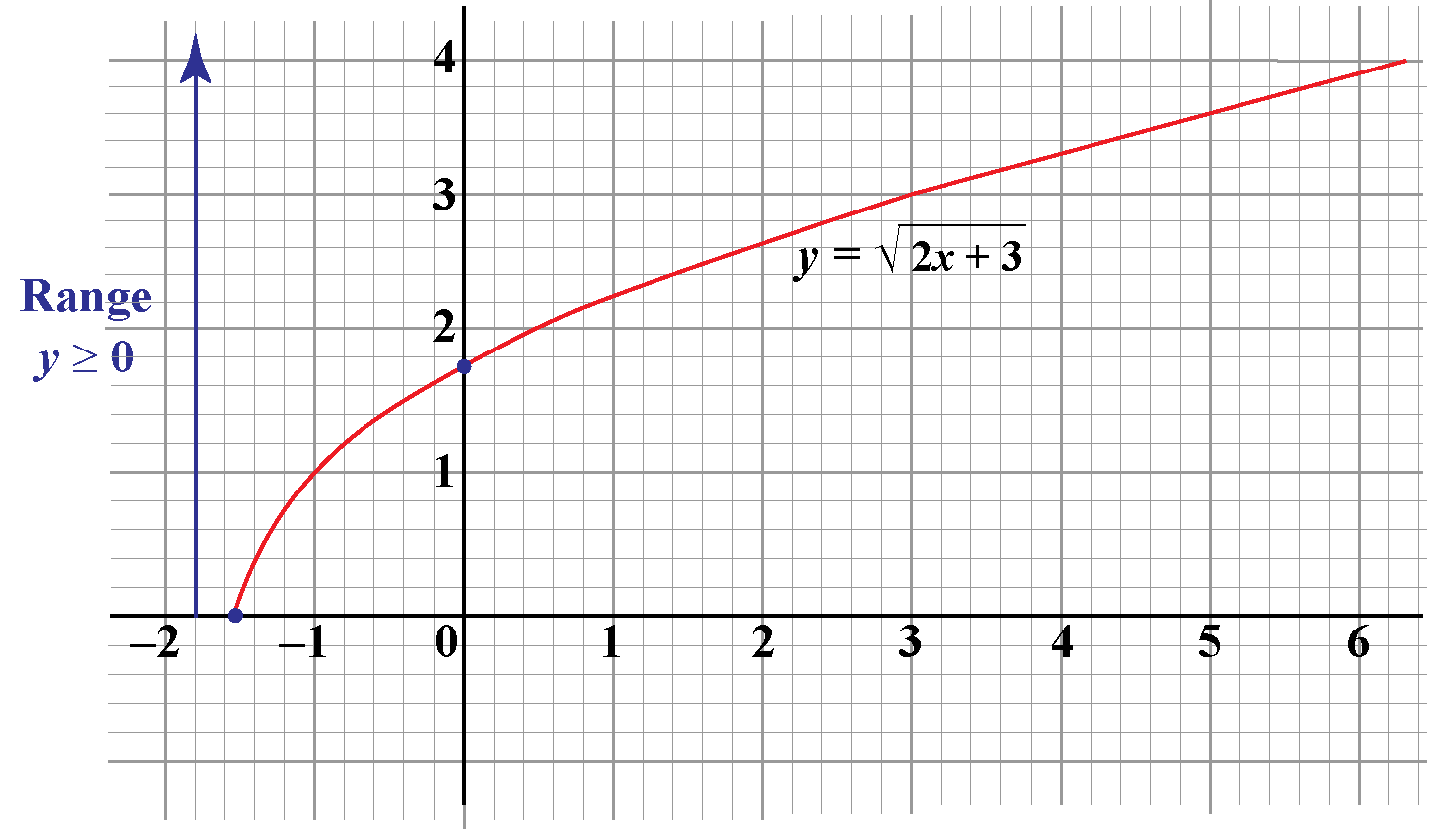### Range of an Absolute Value Function

The absolute value of something always results in a non-negative value.

The y-values of the function $$y=|ax+b|$$ are always non-negative.

Thus, the range of an absolute value function of the form $$y= |ax+b|$$ is:

 $$\{y \in R / y \geq 0\}$$ (OR) $$[0, \infty)$$

Example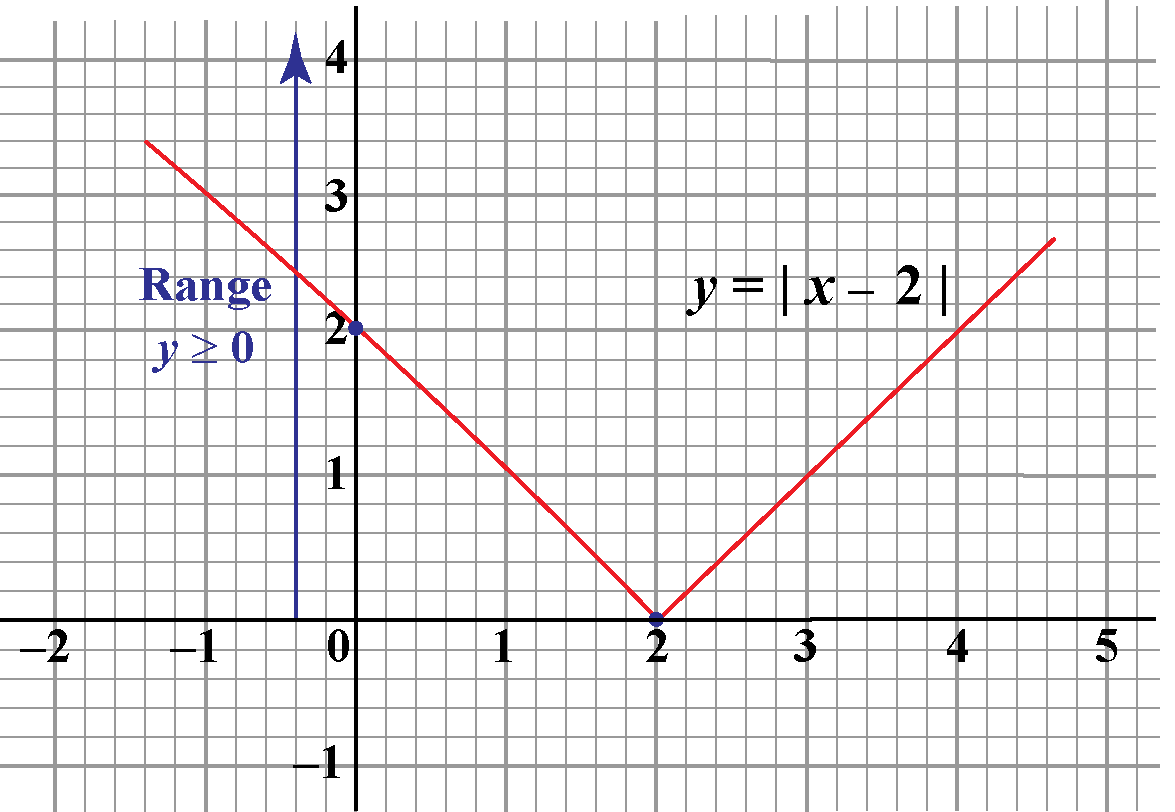## How to Find the Range in Algebra Using Graph?

To find the range of a function using its graph,

• We first graph the function.
• Look for the y-values that are covered by the graph.

We have already seen how to find the ranges of different types of functions using the graph in the above section.

Example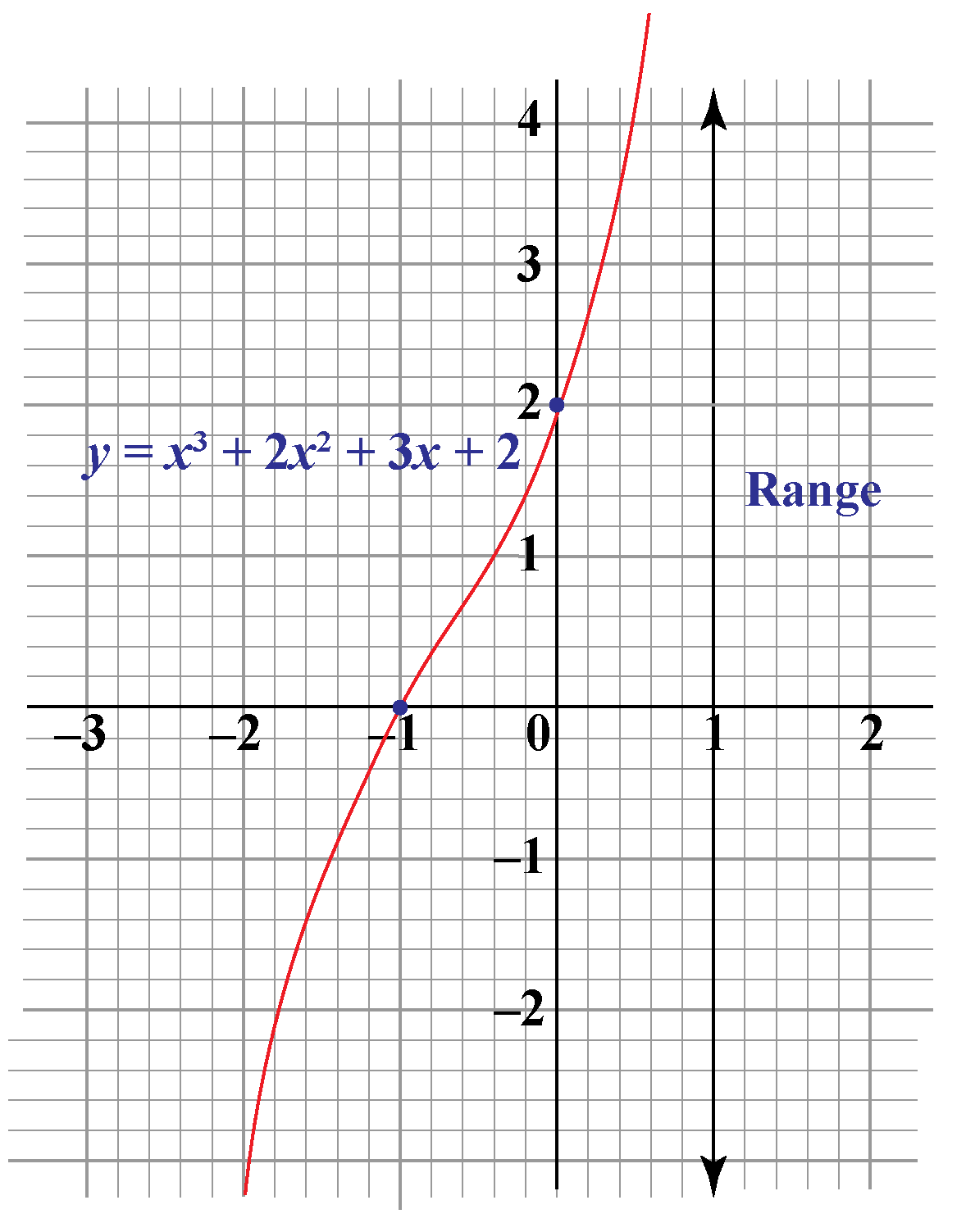From the above graph, the graph of $$y=x^3+2x^2+3x+2$$ is the set of all real numbers, $$R$$.

The following simulation shows how to find the domain (the set of all x-values) and the range (the set of all y-values) of different types of functions after graphing it.Tips and Tricks
1. The easiest method to find the range of function is by graphing it, and looking for the y-values covered by the graph.
2. In case of a square root function (or) an absolute value function, the range is always $$y \geq 0$$
3. To find the range of a quadratic function, it is sufficient to see if it has a maximum or minimum value.
The maximum/minimum value of a quadratic function is the y-coordinate of its vertex.
4. The domain and range of both linear function and cubic function is the set of all real numbers.

## Solved Examples

Here are some examples of domain and range.

 Example 1

Mia is trying to find the domain and range of the function $$y= 2-\sqrt{-3x+2}$$ algebraically. Can we help her?

Solution

Domain

A square root function is defined only when the value inside it is a non-negative number. So for domain,

\begin{align} -3x+2 & \geq 0 \\[0.2cm] -3x & \geq -2\\[0.2cm] x &\leq \dfrac{2}{3} \,[ \text{Divided both sides by -3 }] \end{align}

Range

We already know that the square root function results in a non-negative value always.

$\sqrt{-3x+2} \geq 0\\[0.2cm] \text{Multiply with -1 on both sides}\\[0.2cm] -\sqrt{-3x+2}\ \leq 0 \\[0.2cm] \text{Adding 2 on both sides},\\[0.2cm] 2-\sqrt{-3x+2}\ \leq 2\\[0.2cm] y \leq 2$

Thus,

 Domain = $$\left\{ x \in R/x \leq \dfrac{2}{3} \right\}$$ Range = $$\{y \in R / y \leq 2\}$$
 Example 2

James found the domain of the function $$y= \dfrac{1}{\sqrt x}$$ to be $$x>0$$

But he is stuck with the range of the function. Can we help him?

Also, draw its graph to check whether the answer is correct or not.

Solution

We already know that the square root function results in a non-negative value.

So $$\sqrt x$$ is non-negative for any value of $$x$$ in the domain.

So $$\dfrac{1}{\sqrt x}$$ also must be non-negative.

But $$\dfrac{1}{\sqrt x}$$ cannot be zero for any value of $$x$$.

Thus, $\dfrac{1}{\sqrt x} > 0, \text{ for any x in the domain}$

Thus, the range of the function is,

 $$\{y \in R/ y>0\}$$ (OR) $$(0, \infty)$$

Let's graph the given function and see whether the answer is correct.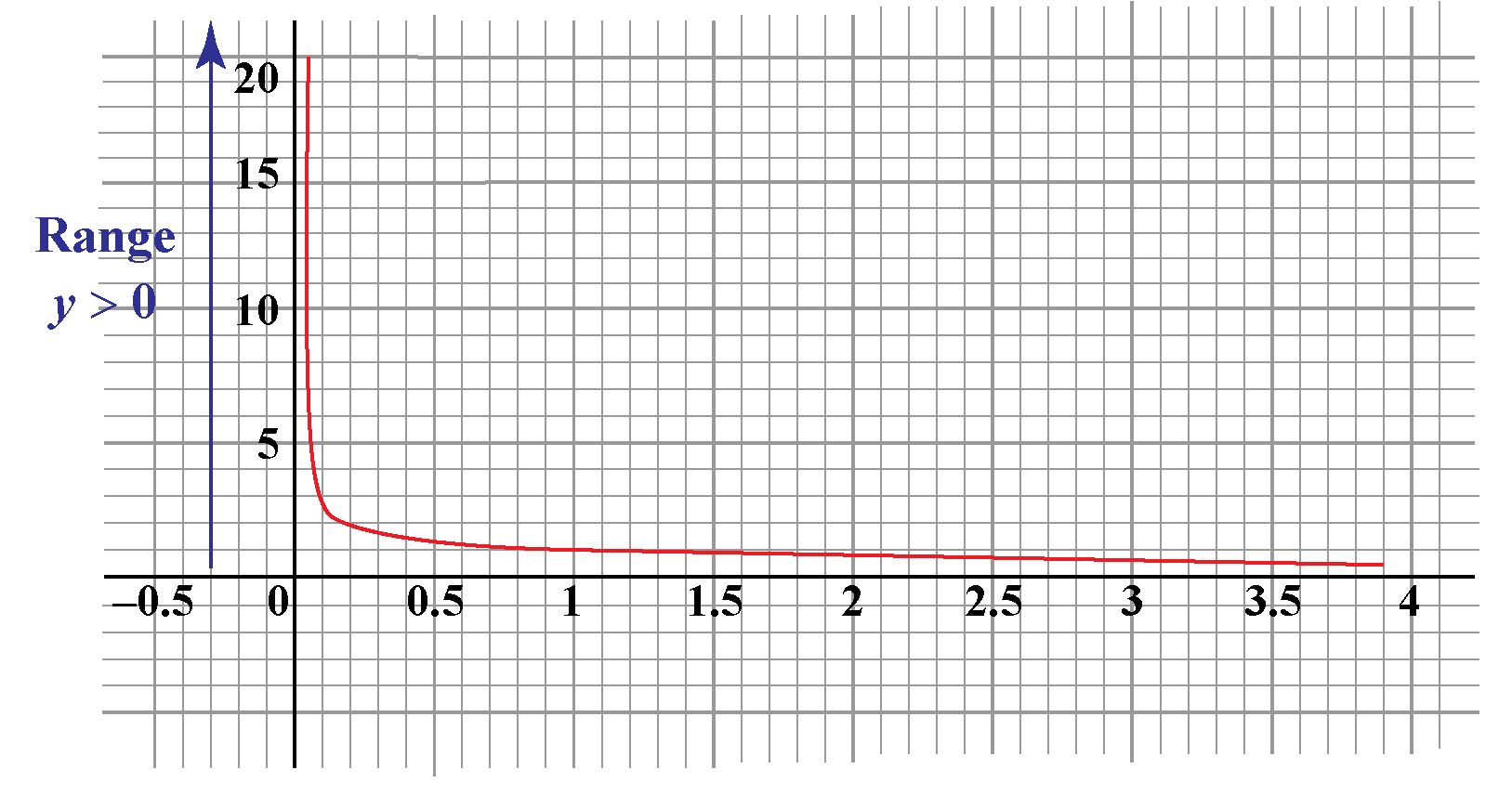Here, the graph covers all values of y above the x-axis except y=0. Thus, the range is $$\{y \in R/ y>0\}$$

## Interactive Questions

Here are a few activities for you to practice.

## Let's Summarize

We hope you enjoyed learning about Range in Algebra with the simulations and practice questions. Now, you will be able to easily solve problems on the range of a function, examples of domain and range, and how to find the range of a function.

At Cuemath, our team of math experts is dedicated to making learning fun for our favorite readers, the students!

Through an interactive and engaging learning-teaching-learning approach, the teachers explore all angles of a topic.

Be it worksheets, online classes, doubt sessions, or any other form of relation, it’s the logical thinking and smart learning approach that we, at Cuemath, believe in.

## 1. How do you write a range?

The range of a function $$y=f(x)$$ is the set of all possible y-values that the function gives.

We write the range as a set or an interval.

## 2. Why is range useful?

The range is useful to decide the scale of the y-axis while graphing a function.

## 3. What is the range of quadratic function?

The range of a quadratic function $$y=a(x-h)^2+k$$ is:

• $$y \geq k$$ if the function has a minimum value, that is, when a>0
• $$y\leq k$$ if the function has a maximum value, that is, when a<0
More Important Topics
Numbers
Algebra
Geometry
Measurement
Money
Data
Trigonometry
Calculus
More Important Topics
Numbers
Algebra
Geometry
Measurement
Money
Data
Trigonometry
Calculus